# Free Maths Worksheets

To view our free maths worksheets simply click the arrows to move through the set and when you see a worksheet you'd like to view in more detail, simply click on the worksheet to see the full size sample.

Please note; You must register with 10ticks to access the full size sample materials. By registering with 10ticks, you will also have two weeks free access to the 10ticks Online Worksheet Licence.

1 / 40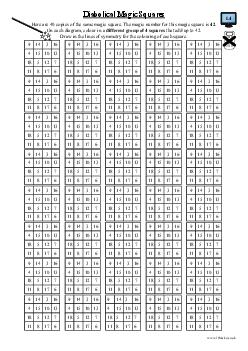Diabolical Magic Square (a)
Number - addition and subtraction
Comment: 4.2.39
2 / 40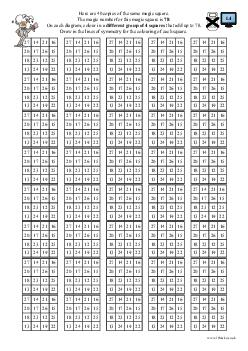Diabolical Magic Square (b)
Number - addition and subtraction
Comment: 4.2.40
3 / 40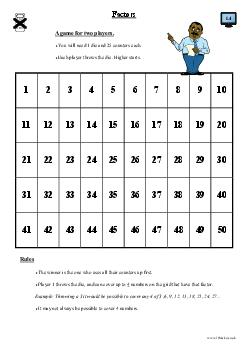Factors
Number - addition, subtraction, multiplication and division
Comment: 4.2.22
4 / 40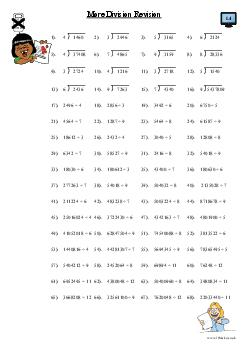More Division Revision
Number - addition, subtraction, multiplication and division
Comment: 4.2.12
5 / 40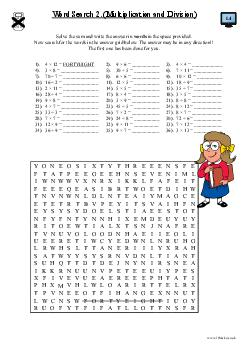Word Search (Multiplication and Division) (b)
Number - multiplication and division
Comment: 4.2.14
6 / 40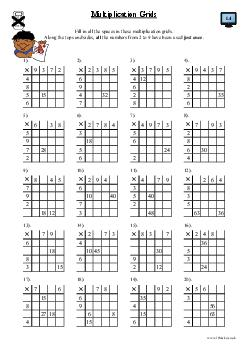Multiplication Grids (a)
Number - multiplication and division
Comment: 4.2.17
7 / 40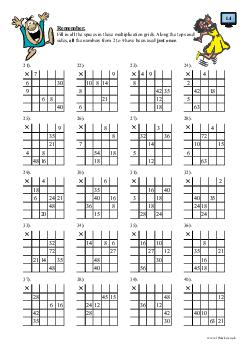Multiplication Grids (b)
Number - multiplication and division
Comment: 4.2.18
8 / 40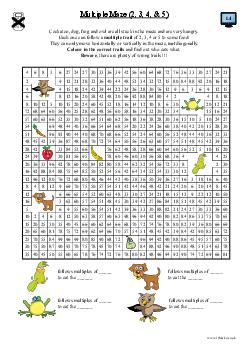Multiple Maze (2, 3, 4 & 5)
Number - multiplication and division
Comment: 4.2.26
9 / 40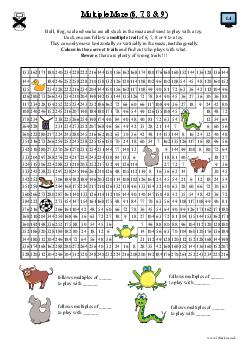Multiple Maze (6, 7, 8 & 9)
Number - multiplication and division
Comment: 4.2.30
10 / 40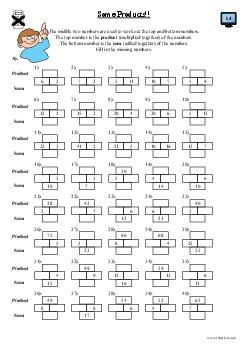Some Products (a)
Number - multiplication and division
Comment: 4.2.33
11 / 40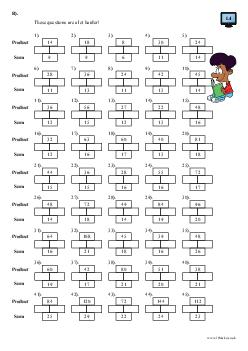Some Products (b)
Number - multiplication and division
Comment: 4.2.34
12 / 40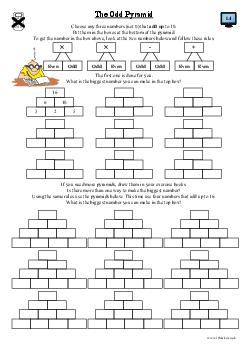The Odd Pyramid (a)
Number - multiplication and division
Comment: 4.2.41
13 / 40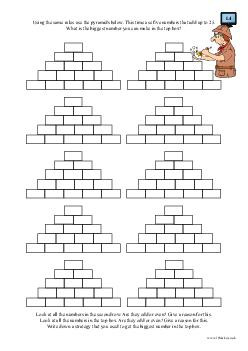The Odd Pyramid (b)
Number - multiplication and division
Comment: 4.2.42
14 / 40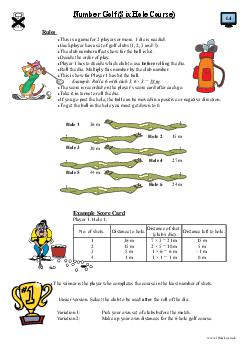Number Golf (Six Hole Course)
Number - multiplication and division
Comment: 4.2.25
15 / 40

Multiplication Puzzles
Number - multiplication and division
Comment: 4.2.31
16 / 40

Triangle
Number - multiplication and division
Comment: 4.2.20
17 / 40

Multiple Maze (3, 4, 5 & 6)
Number - multiplication and division
Comment: 4.2.27
18 / 40

Multiple Maze (4, 5, 6 & 7)
Number - multiplication and division
Comment: 4.2.28
19 / 40

Multiple Maze (5, 6, 7 & 8)
Number - multiplication and division
Comment: 4.2.29
20 / 40

Making a Calculator (Napier's Bones)
Number - multiplication and division
Comment: 4.2.32
21 / 40

Hexagon Puzzle Cards (Addition and Subtraction)
Number - multiplication and division
Comment: 4.2.36
22 / 40

Hexagon Puzzle Cards (Multiplication and Division)
Number - multiplication and division
Comment: 4.2.37
23 / 40

Multiplagons (a)
Number - multiplication and division
Comment: 4.2.15
24 / 40

Multiplagons (b)
Number - multiplication and division
Comment: 4.2.16
25 / 40

Four in a Line - Multiplication Mania
Number - multiplication and division
Comment: 4.2.21
26 / 40

Number Hexagon Puzzle Grid
Number - multiplication and division
Comment: 4.2.35
27 / 40

Hex - A Multiplying Game
Number - multiplication and division
Comment: 4.2.19
28 / 40

Bingo Cards (3 x 3)
Number - multiplication and division
Comment: 4.2.23
29 / 40

Bingo Cards (4 x 3)
Number - multiplication and division
Comment: 4.2.24
30 / 40

Multiplication Grids
Number - multiplication and division
Comment: 4.2.38
31 / 40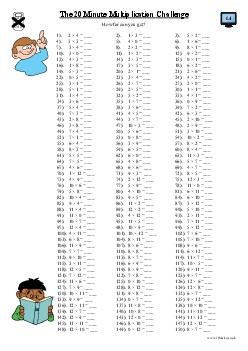The 20 Minute Multiplication Challenge
Number - multiplication and division
Comment: 4.2.5
32 / 40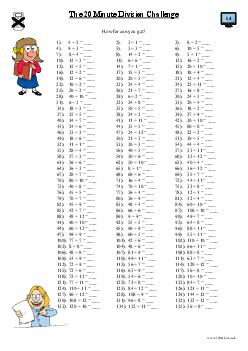The 20 Minute Division challenge
Number - multiplication and division
Comment: 4.2.6
33 / 40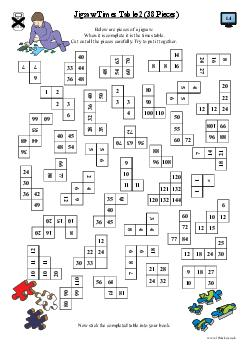Jigsaw Timestable (b)
Number - multiplication and division
Comment: 4.2.4
34 / 40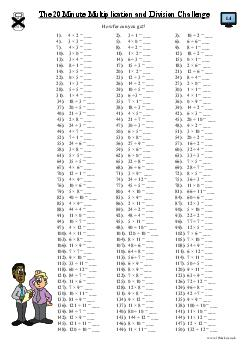The 20 Minute Multiplication and Division Challenge
Number - multiplication and division
Comment: 4.2.11
35 / 40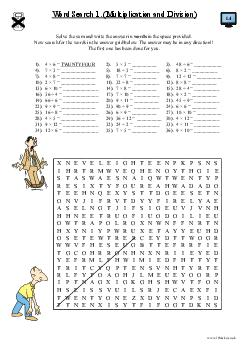Word Search (Multiplication and Division) (a)
Number - multiplication and division
Comment: 4.2.13
36 / 40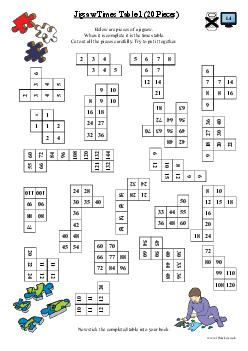Jigsaw Timestable (a)
Number - multiplication and division
Comment: 4.2.3
37 / 40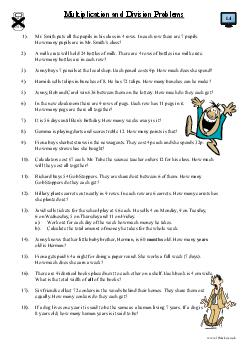Multiplication and Division Problems (a)
Number - multiplication and division
Comment: 4.2.7
38 / 40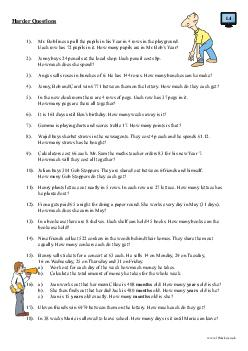Multiplication and Division Problems (b)
Number - multiplication and division
Comment: 4.2.8
39 / 40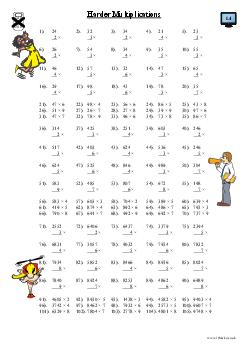Harder Multiplication
Number - multiplication and division
Comment: 4.2.9
40 / 40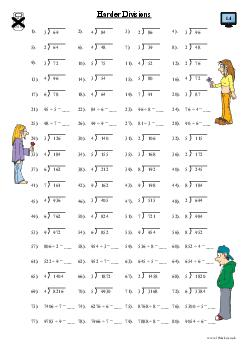Harder Division
Number - multiplication and division
Comment: 4.2.10

Alternatively, to view our Worksheet Sample Packs grouped via Year or our Action Maths, Mastery and GCSE 9 - 1 syllabus samples please click on the appropriate option below;

All contents ©Fisher Educational Ltd 2002. All rights reserved. 10ticks.co.uk is the trading name and Registered trade mark of Fisher Educational Ltd. Registered in England No. 3668099